# Parallel surfaces

(diff) ← Older revision | Latest revision (diff) | Newer revision → (diff)

Diffeomorphic equi-oriented surfacesand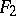having parallel tangent planes at corresponding points and such that the distancebetween corresponding points of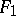andis constant and equal to that between the corresponding tangent planes. The position vectors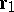and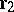of two parallel surfacesandare connected by a relation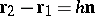, whereis a unit normal vector that is the same foratandat.

Thus, one can define a one-parameter family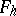of surfaces parallel to a given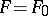, whereis regular for sufficiently small values offor which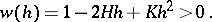To the values of the rootsand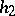of the equation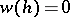there correspond two surfaces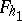and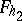that are evolutes of, so that parallel surfaces have a common evolute (cf. Evolute (surface)). The mean curvatureand the Gaussian curvatureof a surfaceparallel toare connected with the corresponding quantitiesandofby the relations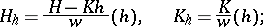lines of curvature of parallel surfaces correspond to each other, so that between them there is a Combescour correspondence, which is a special case of a Peterson correspondence.

For a linear family of closed convex parallel surfaces (depending linearly on a parameter) the Steiner formula holds: The volume of the point set bounded by them is a polynomial of degree 3 in. An analogous result holds for arbitrary dimensions. The Steiner formula is a special case of formulas for general polynomials in Minkowski's theory of mixed volumes, and, even more general, in the theory of valuations.